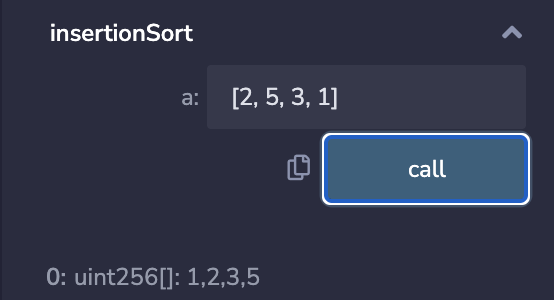# WTF Solidity: 10. Control Flow

Time:
Best Score:

Recently, I have been revisiting Solidity, consolidating the finer details, and writing "WTF Solidity" tutorials for newbies.

Codes and tutorials are open source on GitHub: github.com/AmazingAng/WTFSolidity

In this section, we will introduce control flow in Solidity, and write a insertion sort (`InsertionSort`), a program that looks simple but is actually bug-prone.

## Control Flow​

Solidity's control flow is similar to other languages, mainly including the following components:

1. `if-else`
``function ifElseTest(uint256 _number) public pure returns(bool){    if(_number == 0){    return(true);    }else{    return(false);    }}``
1. `for loop`
``function forLoopTest() public pure returns(uint256){    uint sum = 0;    for(uint i = 0; i < 10; i++){    sum += i;    }    return(sum);}``
1. `while loop`
``function whileTest() public pure returns(uint256){    uint sum = 0;    uint i = 0;    while(i < 10){    sum += i;    i++;    }    return(sum);}``
1. `do-while loop`
``function doWhileTest() public pure returns(uint256){    uint sum = 0;    uint i = 0;    do{    sum += i;    i++;    }while(i < 10);    return(sum);}``
1. Conditional (`ternary`) operator

The `ternary` operator is the only operator in Solidity that accepts three operands：a condition followed by a question mark (`?`), then an expression `x` to execute if the condition is true followed by a colon (`:`), and finally the expression `y` to execute if the condition is false: `condition ? x : y`.

This operator is frequently used as an alternative to an `if-else` statement.

``// ternary/conditional operatorfunction ternaryTest(uint256 x, uint256 y) public pure returns(uint256){    // return the max of x and y    return x >= y ? x: y; }``

In addition, there are `continue` (immediately enter the next loop) and `break` (break out of the current loop) keywords that can be used.

## `Solidity` Implementation of Insertion Sort​

Note: Over 90% of people who write the insertion algorithm with Solidity will get it wrong at the first try.

### Insertion Sort​

The sorting algorithm solves the problem of arranging an unordered set of numbers from small to large, for example, sorting `[2, 5, 3, 1]` to `[1, 2, 3, 5]`. Insertion Sort (`InsertionSort`) is the simplest and first sorting algorithm that most developers learn in their computer science class. The logic of `InsertionSort`:

1. from the beginning of the array `x` to the end, compare the element `x[i]` with the element in front of it `x[i-1]`; if `x[i]` is smaller, switch their positions, compare it with `x[i-2]`, and continue this process.

The schematic of insertion sort:### Python Implementation​

Let's first look at the Python Implementation of the insertion sort：

``# Python program for implementation of Insertion Sortdef insertionSort(arr):    for i in range(1, len(arr)):        key = arr[i]        j = i-1        while j >=0 and key < arr[j] :                arr[j+1] = arr[j]                j -= 1        arr[j+1] = key    return arr``

### Solidity Implementation (with Bug)​

Python version of Insertion Sort takes up 9 lines. Let's rewrite it into Solidity by replacing `functions`, `variables`, and `loops` with solidity syntax accordingly. It only takes up 9 lines of code:

``    // Insertion Sort (Wrong version）    function insertionSortWrong(uint[] memory a) public pure returns(uint[] memory) {        for (uint i = 1;i < a.length;i++){            uint temp = a[i];            uint j=i-1;            while( (j >= 0) && (temp < a[j])){                a[j+1] = a[j];                j--;            }            a[j+1] = temp;        }        return(a);    }``

But when we compile the modified version and try to sort `[2, 5, 3, 1]`. BOOM! There are bugs! After 3-hour debugging, I still could not find where the bug was. I googled "Solidity insertion sort", and found that all the insertion algorithms written with Solidity are all wrong, such as: Sorting in Solidity without Comparison

Errors occurred in `Remix decoded output`:### Solidity Implementation (Correct)​

With the help of a friend from `Dapp-Learning` community, we finally found the problem. The most commonly used variable type in Solidity is `uint`, which represent a non-negative integer. If it takes a negative value, we will encounter an `underflow` error. In the above code, the variable `j` will get `-1`, causing the bug.

So, we need to add `1` to `j` so it can never take a negative value. The correct insertion sort solidity code:

``    // Insertion Sort（Correct Version）    function insertionSort(uint[] memory a) public pure returns(uint[] memory) {        // note that uint can not take negative value        for (uint i = 1;i < a.length;i++){            uint temp = a[i];            uint j=i;            while( (j >= 1) && (temp < a[j-1])){                a[j] = a[j-1];                j--;            }            a[j] = temp;        }        return(a);    }``

Result:## Summary​

In this lecture, we introduced control flow in Solidity and wrote a simple but bug-prone sorting algorithm. Solidity looks simple but have many traps. Every month, projects get hacked and lose millions of dollars because of small bugs in the smart contract. To write a safe contract, we need to master the basics of the Solidity and keep practicing.Products
Community
Donation